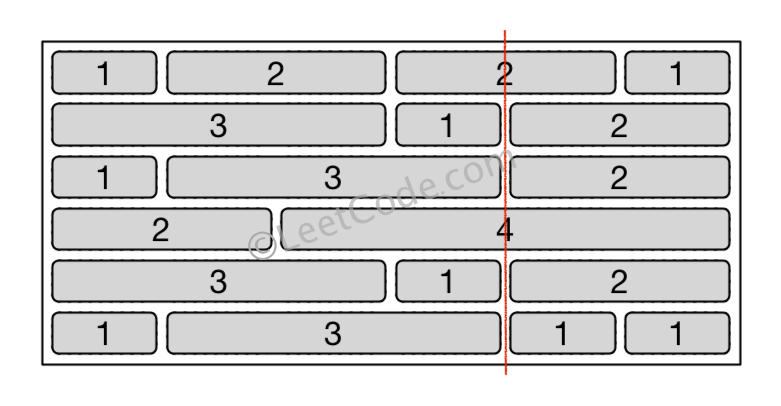554. Brick Wall

There is a brick wall in front of you. The wall is rectangular and has several rows of bricks. The bricks have the same height but different width. You want to draw a vertical line from the top to the bottom and cross the least bricks.

The brick wall is represented by a list of rows. Each row is a list of integers representing the width of each brick in this row from left to right.

If your line go through the edge of a brick, then the brick is not considered as crossed. You need to find out how to draw the line to cross the least bricks and return the number of crossed bricks.

You cannot draw a line just along one of the two vertical edges of the wall, in which case the line will obviously cross no bricks.

Example:

```Input: [[1,2,2,1],
[3,1,2],
[1,3,2],
[2,4],
[3,1,2],
[1,3,1,1]]

Output: 2

Explanation:```

Note:

1. The width sum of bricks in different rows are the same and won't exceed INT_MAX.
2. The number of bricks in each row is in range [1,10,000]. The height of wall is in range [1,10,000]. Total number of bricks of the wall won't exceed 20,000.

554. Brick Wall
``````struct Solution;
use std::collections::HashMap;

impl Solution {
fn least_bricks(wall: Vec<Vec<i32>>) -> i32 {
let n = wall.len();
let w: i32 = wall.iter().sum();
let mut hm: HashMap<i32, usize> = HashMap::new();
for row in wall {
let mut sum = 0;
for x in row {
sum += x;
*hm.entry(sum).or_default() += 1;
}
}
let mut max = 0;
for (k, v) in hm {
if k != w {
max = max.max(v);
}
}
(n - max) as i32
}
}

#[test]
fn test() {
let wall = vec_vec_i32![
[1, 2, 2, 1],
[3, 1, 2],
[1, 3, 2],
[2, 4],
[3, 1, 2],
[1, 3, 1, 1]
];
let res = 2;
assert_eq!(Solution::least_bricks(wall), res);
}
``````